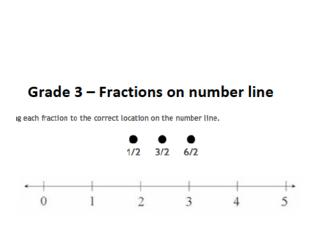Download Presentation# Link to Content and Practices - PowerPoint PPT Presentation

Download Presentation##### Link to Content and Practices

Download Policy: Content on the Website is provided to you AS IS for your information and personal use and may not be sold / licensed / shared on other websites without getting consent from its author. While downloading, if for some reason you are not able to download a presentation, the publisher may have deleted the file from their server.

- - - - - - - - - - - - - - - - - - - - - - - - - - - E N D - - - - - - - - - - - - - - - - - - - - - - - - - - -
##### Presentation Transcript

1. Link to Content and Practices 3.NF.A.2. Understand a fraction as a number on the number line; represent fractions on a number line diagram. a. Represent a fraction 1/b on a number line diagram by defining the interval from 0 to 1 as the whole and partitioning it into b equal parts. Recognize that each part has size 1/b and that the endpoint of the part based at 0 locates the number 1/b on the number line. b. Represent a fraction a/b on a number line by marking off lengths 1/b from 0. Recognize that the resulting interval has size a/b and that its endpoint locates the number a/b on the number line. MP.7 (Look for and make use of structure). This task requires students to attend to the parts of each fraction expression (numerators and denominators); for example, if students notice that all of the denominators are the same then they may be able to complete the task more efficiently. Visual fraction models, like the number line, are an important tool. (MP.5, Use appropriate tools strategically). Although this item does not require the student to make an independent/strategic decision to use a number line, as it appears in the prompt.

2. Task Prerequisites • Before you would expect your students to successfully complete this task they would need to have experiences using a number line. This would include representing a fraction on a number line with denominators 2, 3, 4, 6, and 8. For example, where would you place ½ on a number line below (note provide 0-1 number line).

3. Using this task in your classroom • There are many ways that this task or similar ones that you might develop could be used to support your teaching. Consider that this task involves the number line and comparing and ordering fractions, in this case halves. Students will need to technologically drag and drop 1/2, 3/2, and 6/2. As they complete this activity you might consider asking the following: • Which of these fractions is the least amount? Greatest? How do you know? • What can you tell me about these three fractions? • How did you decide where to drag and drop or place the fractions on the number line?

4. Next Steps • Provide the students with number lines from 0-5. Ask them to compare, order, and place the following fractions on the number line: 3/4, 1/4, 4/4, 8/4, and 12/4. • Extension Activity (for Advanced Students) • What if we just changed this fractions and they were 2/1, 2/3, and 2/6. Now what? Where would you place them on the number line? • How did you decide where to place them?

5. All PARCC and Smarter Balanced sample items • Tasks adapted from the Illustrative Mathematics Project (McCallum site) • Thinking: • Coverage based on PARCC – green, blue, and yellow dots • Coverage of all Content Domains • Items for each grade level: grades 3, 4 and 5

6. Thoughts?